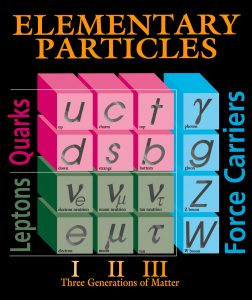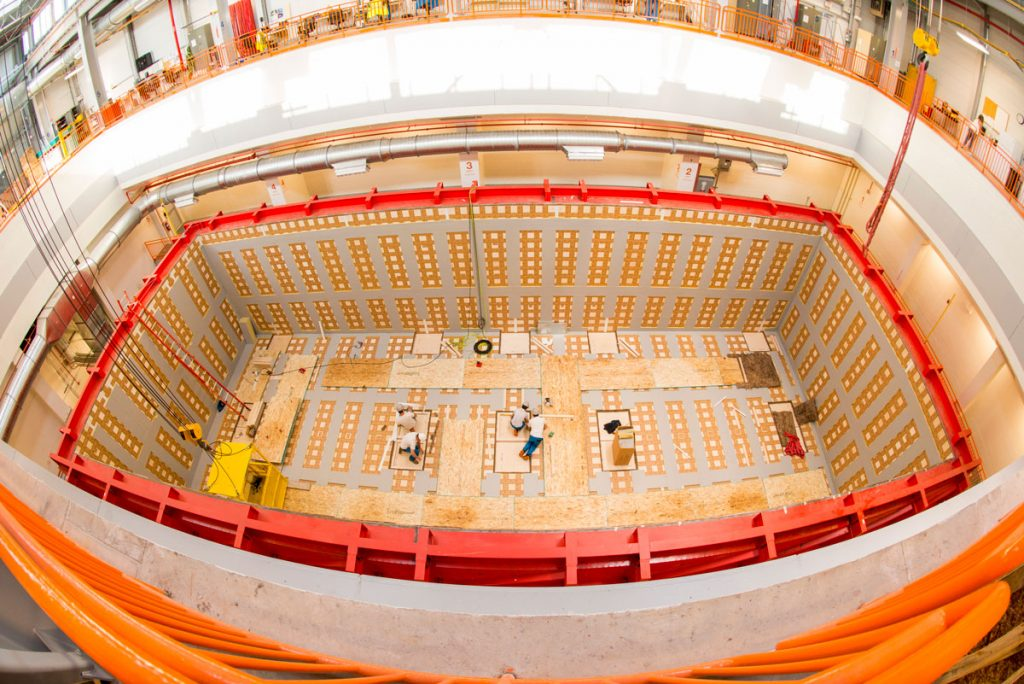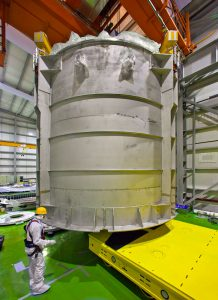# How many flavors of neutrinos are there?The basic building blocks are the six leptons, six quarks, four force carriers, and the Higgs boson. Credit: Fermilab

Particles come in different flavors.

For example, there are three kinds of charged leptons, the lightest of which is the well-known electron that helps power your life. There are six kinds of quarks, two of which are found in the protons and neutrons that make up the screen you are using to read this. But things are always trickier with neutrinos. So just how many kinds of neutrinos are there?

Scientists know about three neutrino flavors (the electron neutrino, muon neutrino, and tau neutrino) so far, which are related to the three charged lepton flavors (the electron, muon and tau). But there could be additional flavors of neutrinos—for example, the proposed “sterile” neutrinos that would interact with matter only through gravity and could come in different varieties as well. Researchers aren’t sure if there are zero, one, or a bunch of these sterile neutrinos.

Sterile neutrinos wouldn’t interact the same way that normal neutrinos do, through the weak force, making them even harder to see than regular (so-called “active”) neutrinos. After all, if you have a particle that interacts only through gravity and is as light as the neutrino, it will be practically invisible.

But because the standard flavors of neutrinos might oscillate, or change, into sterile neutrinos, scientists might still get a glimpse of the sneaky particles. If a muon or electron neutrino has to “oscillate” through a sterile phase, it could change the rate at which electron neutrinos appear. This is exactly the kind of anomaly that scientists have seen in some experiments; there were more electron neutrinos appearing than expected based on what researchers knew of neutrinos.

These anomalies point to an ideal distance to search for sterile neutrinos. That distance is typically a few hundred meters for accelerator experiments, meaning detectors can be built fairly close to the source of neutrinos—though longer-distance experiments many hundreds of kilometers away could also see evidence for sterile neutrinos. Many experiments are currently looking for them, including a suite of three experiments that make up Fermilab’s Short-Baseline Neutrino Program.The ICARUS detector, which is one of three experiments that make up the Fermilab Short-Baseline Neutrino Program, will sit inside a large cooler at Fermilab. ICARUS was part of a long-baseline neutrino experiment in Italy and was refurbished at CERN before coming to the United States to start its next hunt for neutrinos. Credit: ICARUS collaboration / Fermilab

You might wonder why scientists aren’t certain how many types of neutrinos there are, especially when they do know how many kinds of quarks and leptons exist. It stems from some experimental measurements that don’t fit into the three-neutrino picture. To try to explain these strange measurements, scientists introduced an additional neutrino.

But let’s take a step back and work through why scientists think there are three different mass neutrinos to start with.A detector tank, prior to installation at the Daya Bay Reactor Neutrino Experiment. Reactor experiments gave physicists vital information about neutrinos. Credit: Roy Kaltschmidt / Berkeley Lab

If there are three neutrinos that all have different masses, then there can only be two independent mass differences: the small mass difference between the two neutrinos that are close in weight (ν1 and ν2) and the large mass difference—the difference between the outlier mass neutrino (ν3) that is much heavier or much lighter and its nearest neighbor. The third mass difference is equal to the sum of the other two mass differences. You can think of it like a number line. The difference between 1 and 4 is 3, the difference between 4 and 10 is 6, and the difference between 1 and 10 is 3+6.

By looking at how neutrinos oscillate, scientists have been able to pin down the small mass difference very well. The best measurement of that mass difference comes from looking at the energies of neutrinos that come from reactors, usually carrying energies of a few million electronvolts, that are about 200 kilometers away from their source.

Scientists have been able to pin down the large mass difference very well, too. The best measurement of that mass difference comes from looking at the energies of neutrinos that come from accelerator sources. Those neutrinos have on the order of a billion or a few billion electronvolts, and the detectors are typically located 300 to 800 kilometers away from the source.

Scientists have a prediction for the likelihood of one of those neutrinos staying the same flavor. In a simpler picture, where there is only one mass difference (or two neutrinos), it looks like this:

P = 1 – sin2 (1.27δm2 L/E)

In this equation, P is the probability the neutrino will keep its original flavor, L is the distance (in kilometers) between where the neutrino was produced and where it was detected, E is the neutrino energy (in billions of electronvolts), and δm2 is the difference between the two different neutrino masses squared (in squared electronvolts).

Scientists looked at the prediction to see whether it would hold true for both the reactor and the accelerator measurements if each experiment were measuring only one mass difference. But as we saw earlier, the energies—E—from the two measurements are very different. The accelerator neutrinos had a thousand times more energy than the reactor neutrinos—millions versus billions of electronvolts. Those two wildly different values of E produce two very different values for P. And if you have two different values for P—the probability that a neutrino keeps its original flavor—then you have two different flavors for the original flavor to change into. And the only way for that to work is to have three different masses of neutrino: the original and the two it would oscillate into. This is one of the pieces of evidence that leads scientists to conclude that there are three different mass neutrinos.

Scientists have observed the “disappearance” of a neutrino flavor. They might send 300 electron neutrinos to a detector and witness the arrival of only 100. If one flavor of neutrino is “disappearing” in a detector, then another neutrino flavor should be appearing in its place. Otherwise, where are those pesky, disappearing neutrinos going? And in fact, this is what scientists found. Using neutrino beams from accelerators, experimenters saw electron-flavored neutrinos appearing in the beam at precisely the same energy where the muon-flavored neutrinos were disappearing.MiniBooNE was an experiment at Fermilab that used a steel tank filled with photomultiplier tubes and mineral oil. It followed up on the hints of sterile neutrinos seen at Los Alamos National Laboratory’s LSND experiment. Credit: Fermilab

But here’s where the fourth (or fifth, or other) neutrinos come in.

Imagine, knowing what you now know, that you see electron neutrinos appearing in a muon beam at a much smaller distance/energy combination — a smaller L/E — than anything scientists have set up before. If you do the math, you see that a smaller L/E implies a much larger mass difference than either the large or small mass differences we’ve described. One way to make sense of that is to introduce yet another mass of neutrino, and make the mass difference between that neutrino and the other ones we know about much larger, and defined by the new distance/energy combination.

This scenario has happened two different times now in experiments called LSND, the Liquid Scintillator Neutrino Detector at Los Alamos National Laboratory, and MiniBooNE at Fermilab. However, the electron neutrino excess didn’t happen at exactly the same distance/energy combinations in the two cases. If you interpret each of these experimental results as oscillations, then there could be more than three mass neutrinos whizzing through our universe.

Given that experiments have seen these anomalies, why do neutrino scientists still paint a three-neutrino picture? One reason is related to a very heavy force particle called the Z boson. By looking at all the different ways that this particle decays, scientists can predict how often it should decay into neutrinos. Given the theory of Z boson decays and how often the particle has been measured to decay into neutrinos, scientists know that there are only three flavors of neutrinos into which the Z can decay.

That means if scientists do introduce a fourth (or fifth, or 20th) neutrino mass, then they also introduce a fourth (or fifth, or 20th) flavor. Then, they have to come up with some reason for that flavor to NOT care about the Z boson like the other neutrinos do. For the extra flavors that come along for the ride, the Z boson would have to be invisible, making the extra flavors a kind of “sterile” or noninteracting neutrino.

The jury is still out on whether or not there are more than three neutrinos in the universe, and a variety of experiments are under way to try to confirm or refute any of these new neutrinos.